# Modal LogicExamples

For convenience, we reproduce the item Logic/Modal Logic of Principia Metaphysica in which the modal logic is defined: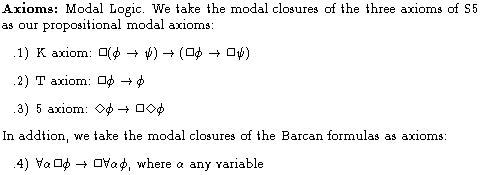In this tutorial, we give examples of the axioms, consider some rules of inference (and in particular, the derived Rule of Necessitation), and then draw out some consequences.

To help us reason with modal notions, we may intuitively appeal to the notion of a possible world, i.e., a way things might have gone. Our world is just one possible world of an infinite number of such worlds. For now, let us suppose this idea is well-understood (in Principia Metaphysica it can be precisely defined). Using the notion of a possible world, we may intuitively think of the claim: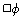as the claim: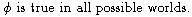And we may intuitively think of the claim: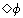as the claim: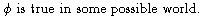We will exploit these intuitions in what follows to help us to get a picture of (i.e., intuitive model of) the axioms.

## Examples of the Axioms and Rules

Instance of Axiom 1:Recall that the predicate A!x asserts that x is abstract. Suppose that Rx asserts that x is round. Then this instance of Axiom 1 asserts If the conditional, if x is abstract then x is not round, is necessary, then if it is necessary that x is abstract, it is necessary that x is not round . It is in fact an auxiliary hypothesis that abstract objects are not round, and moreover, this is a necessary fact. Moreover, it will be provable that abstract objects are necessarily abstract. So, from this instance of Axiom 1, we should be able to derive that an abstract object x necessarily fails to be round.

Instance of Axiom 2:This simply asserts that if it is necessary that x encodes F, then x encodes F . Intuitively, any statement true at all possible worlds is true (at the actual world).

Instance of Axiom 3: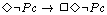Let the predicate P denote the property of being a politician. Let the constant c denote Clinton. Then this instance of Axiom 3 asserts if Clinton might not have been a politician, then it is necessary that he might not have been a politician. Intuitively, this axiom just tells us that statements having the weakest modal status, namely, being possibly true, have that status necessarily. From this axiom, we shall be able to derive the fact that statements having the strongest modal status, namely, being necessarily, true, also have that status necessarily.

Instances of Axiom 4: Barcan Formulas:The first example asserts if everything is necessarily such that it exemplifies P, then it is necessary that everything exemplifies P. Intuitively, this axiom tells us that everything that exists in the domain of quantification at other possible worlds also exists in the domain of quantification of our actual world. If this were not true, then it wouldn't follow from the claim that everything exemplifies property P necessarily that necessarily, everything exemplifies P, for there might be some object at some other possible world (not falling under the range of our quantifier eveything) which failed to exemplify P at that world. In such a circumstance, the antecedent of this axiom would be true, but the conclusion false. Similarly, the second example asserts if every property F is necessarily such that a exemplifies F, then it is necessary that a exemplifies every property F. Intuitively, all the properties that fall in the range of the quantifier at other possible worlds already fall in the range of the quantifier at this possible world.

## Metatheorems and Derived Rules

Metatheorem: Derived Rule of Necessitation (RN): For convenience, we reproduce the item Logic/Rule of Necessitation from Principia Metaphysica:This is the most important rule of inference in modal logic. It basically asserts that anything derivable from necessary truths is a necessary truth. With the exception of the logical axiom governing definite descriptions, all of the logical axioms of our system are necessary truths (the explanation for this will be given in the tutorial on the logic of definite descriptions). So anything derivable from the necessary (`modal') logical axioms alone is a necessary truth. Moreover, if we can derive any consequence that depends solely on modal axioms or added premises which are themselves necessary truths, then such a consequence is also a necessary truth.

In the usual formulation of modal logic, the Rule of Necessitation is taken as a primitive rule of inference. The axioms of the system do not include the modal closures of the logical axioms. In such formulations, it is assumed that all of the logical axioms are necessary truths. So anything derivable solely from such necessary truths will themselves be necessary, and so RN never takes us from truth to falsehood. However, when formulating a modal logic in which one of the logical axioms is not a necessary truth, RN must be restricted. It works normally in any proof where the conclusion rests only on logical axioms that are necessary, but when the conclusion rests on a logical axiom that is not necessary, the application of RN is invalid. For example, on one construal, the conditional claim if it is actually the case that p (is true), then p (is true) is a logical truth but not a necessary truth. This conditional is logically true because it is part of the very meaning of the operator it is actually the case that that any proposition it applies to is true. However, to see that it is not a necessary truth suppose that p is true in the actual world and consider a different world where p is false. At that world, call it w, the antecedent, it is actually the case that p is true, is still true there, because on this construal, the operator it is actually the case that looks back to the actual world to see whether p is true (and since, by hypothesis, we are assuming that p is true at the actual world, it is actually the case that p is true at world w). But the consequence p is true is false at world w, by hypothesis. Since there is a possible world, namely, w, where our conditional is false, it is not a necessary truth. So we do not want to be able to use the Rule of Necessitation upon the logical truth if it is actually the case that p, then p, because this is not a necessary truth. Any system that allows such an operator has to restrict RN.

But modal logic can easily accomodate such contingent, nonmodal logical axioms and still remain classical. The technique is simply to include as axioms all of the modal closures of the `modal' logical axioms. Then the Rule of Necessitation can be derived. The proof is in Principia Metaphysica (in the Appendix, look for the item number that currently corresponds to the item Logic/Rule of Necessitation). RN behaves classically in cases where the derivation involves only necessary truths. Notice that our formulation of RN allows us to apply the rule to consequences derived from non-logical premises, as long as those non-logical premises are also necessary. We may not apply the rule to consequences of contingent premises. For example, it is a contingent fact that Clinton is a politician. But we may not use RN to give us a proof of Necessarily, Clinton is a politician from the contingent premise Clinton is a politician.

Derived Theorem: Rule K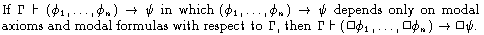Notice that Rule K reduces to RN when n=0.

## Some Further Consequences:

Theorem: Modal Modus PonensThis theorem grounds a plan of reasoning often found in natural deduction systems of modal logic. The reasoning proceeds as follows:
Consider an arbitrary world w. Since if p then q is a necessary truth, it is true at all possible worlds, and so it is true at w. Since p is also a necessary truth, it too is true at all possible worlds and so is true at w. But since both if p then q and p are true at w, q is true at w (since the modal laws of logic apply to every possible world. But we have just proved that q is true at an arbitrarily chosen possible world. So q is true at every possible world, and so is a necessary truth. Thus Necessarily q.
The present theorem underlies and grounds this kind of reasoning.

It is easy to prove Modal Modus Ponens, given Axiom 1 of modal logic. Consider the following sequence of formulas: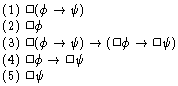This is a proof because: (a) lines 1 and 2 are members of the premise set, (b) line 3 is an instance of Axiom 1 of modal logic, (c) line 4 results from lines 1 and 3 by MP, and (d) line 5 results from lines 2 and 4 by MP.

(Logical) Theorems:The first theorem asserts that we may prove Possibly q from the premises Necessarily, if p then q and Possibly p. It allows us to reason informally as follows:
Given the premise Possibly p, we know that p is true at some possible world. So pick an arbitrary world where p is true (we know there must be at least one) and call it w. Since our other premise is Necessarily, if p then q, we know that the conditional if p then q is true at every possible world, and in particular, it is true at w. But since both if p then q and p are true at w, q is true at w. Since w was an arbitrarily chosen world, we know that from the point of view of the actual world that q is true at some possible world. So Possibly q.
The first theorem, therefore, guarantees the validity of this sort of reasoning. From it, we may derive the logical theorem listed immediately below it, by using the Deduction Theorem.

Logical Theorem: Converse Barcan Formula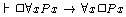Instances of this logical theorem schema assert: if it is necessary that everything has a certain property, then everything is necessarily such that it has that property. Intuitively, this theorem tells us that everything that exists in the domain of quantification of our actual world also exists in the domain of quantification at other possible worlds. If this were not true, then it wouldn't follow from the claim that necessarily, everything exemplifies P that everything exemplifies property P necessarily, for it might be that every object exemplifies P at every world, but some object exemplifying P at our actual world both fails to be in the domain of quantification at some other possible world and fails to exemplify P at that world. In such a circumstance, the antecedent of this theorem would be true, but the conclusion false.

Since Axiom 4 tells us that the domains of quantification at other possible worlds do not increase in size over (i.e., do not contain objects not already in) the domain of quantification at the actual world, and the present theorem tells us that the domain of quantification at other possible worlds do not decrease in size from the domain of the actual world, we know that the domain of quantification stays fixed from world to world. This `fixed domain' of quantification is the simplest kind of quantified modal logic.

Logical Theorems: Equivalent Expression of the Barcan FormulaThis is an equivalent way of expressing the Barcan Formula for objects. It asserts: if it is possible that something exemplifies P, then something possibly exemplifies P. For example, consider the true claim: Clinton might have had a sister. This theorem tells us: if Clinton might have had a sister, then there is something which is such that it might have been Clinton's sister. By MP, we can conclude there is something which is such that it might have been Clinton's sister. Given certain facts about the origin of ordinary concrete objects, namely, that their genetic makeup is essential to them, many philosophers believe that no concrete ordinary object could have been Clinton's sister. But since we have just concluded that some object is such that it might have been Clinton's sister, one wonders which object it is. In the present theory, the answer is: it is a nonconcrete object which might have been concrete. This is not a abstract object, for by definition, an abstract object is not the kind of thing that could be concrete, i.e., not the kind of thing that could have a spatiotemporal location. Rather, this is an object that is not concrete, but which is concrete at some other possible world. Just as you, me, your computer, and other contingent, concrete objects are concrete objects at some possible worlds and but not concrete at others, the objects we are now considering are concrete at other possible worlds but not concrete at our world. Since we know that there is at least one object that, at some other possible world, is Clinton's sister there, let us call the object b. We can say that at any world where b is Clinton's sister, b is concrete at that world. That is because, necessarily, if something is the sister of something else, then both things are concrete.